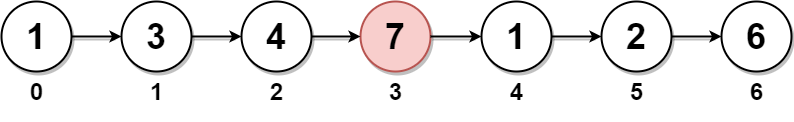# 2095. Delete the Middle Node of a Linked List

Medium
You are given the `head` of a linked list. Delete the middle node, and return the `head` of the modified linked list.
The middle node of a linked list of size `n` is the `⌊n / 2⌋th` node from the start using 0-based indexing, where `⌊x⌋` denotes the largest integer less than or equal to `x`.
• For `n` = `1`, `2`, `3`, `4`, and `5`, the middle nodes are `0`, `1`, `1`, `2`, and `2`, respectively.
Example 1:Output:
[1,3,4,1,2,6]
Explanation:
The above figure represents the given linked list. The indices of the nodes are written below.
Since n = 7, node 3 with value 7 is the middle node, which is marked in red.
We return the new list after removing this node.
Example 2:Output:
[1,2,4]
Explanation:
The above figure represents the given linked list.
For n = 4, node 2 with value 3 is the middle node, which is marked in red.
Example 3:Output:

Explanation:
The above figure represents the given linked list.
For n = 2, node 1 with value 1 is the middle node, which is marked in red.
Node 0 with value 2 is the only node remaining after removing node 1.
Constraints:
• The number of nodes in the list is in the range `[1, 105]`.
• `1 <= Node.val <= 105`

### 解題

/**
* type ListNode struct {
* Val int
* Next *ListNode
* }
*/